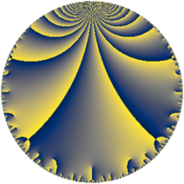# Properties

 Label 50.6.dLevel $50$ Weight $6$ Character orbit 50.d Rep. character $\chi_{50}(11,\cdot)$ Character field $\Q(\zeta_{5})$ Dimension $52$ Newform subspaces $2$ Sturm bound $45$ Trace bound $1$

# Related objects

## Defining parameters

 Level: $$N$$ $$=$$ $$50 = 2 \cdot 5^{2}$$ Weight: $$k$$ $$=$$ $$6$$ Character orbit: $$[\chi]$$ $$=$$ 50.d (of order $$5$$ and degree $$4$$) Character conductor: $$\operatorname{cond}(\chi)$$ $$=$$ $$25$$ Character field: $$\Q(\zeta_{5})$$ Newform subspaces: $$2$$ Sturm bound: $$45$$ Trace bound: $$1$$ Distinguishing $$T_p$$: $$3$$

## Dimensions

The following table gives the dimensions of various subspaces of $$M_{6}(50, [\chi])$$.

Total New Old
Modular forms 156 52 104
Cusp forms 140 52 88
Eisenstein series 16 0 16

## Trace form

 $$52q + 4q^{2} - 4q^{3} - 208q^{4} - 265q^{5} - 72q^{6} + 312q^{7} + 64q^{8} - 1339q^{9} + O(q^{10})$$ $$52q + 4q^{2} - 4q^{3} - 208q^{4} - 265q^{5} - 72q^{6} + 312q^{7} + 64q^{8} - 1339q^{9} + 100q^{10} - 716q^{11} + 96q^{12} - 114q^{13} - 784q^{14} + 120q^{15} - 3328q^{16} + 992q^{17} - 4208q^{18} - 5990q^{19} + 1280q^{20} + 4344q^{21} + 1408q^{22} + 2886q^{23} + 4608q^{24} + 1675q^{25} + 11448q^{26} - 17260q^{27} + 1952q^{28} - 14220q^{29} - 15280q^{30} + 18834q^{31} - 4096q^{32} + 17882q^{33} - 6604q^{34} + 32410q^{35} - 21424q^{36} + 43967q^{37} + 17360q^{38} + 13432q^{39} + 1600q^{40} - 16196q^{41} + 32168q^{42} - 73124q^{43} + 1184q^{44} - 73405q^{45} - 28672q^{46} + 39822q^{47} + 1536q^{48} + 185444q^{49} + 2500q^{50} + 85984q^{51} - 1824q^{52} + 45501q^{53} + 16080q^{54} - 1450q^{55} - 12544q^{56} - 254880q^{57} - 37320q^{58} + 18000q^{59} + 33920q^{60} - 48136q^{61} + 48128q^{62} - 302704q^{63} - 53248q^{64} - 142045q^{65} + 52256q^{66} - 156718q^{67} - 100128q^{68} + 60692q^{69} - 132720q^{70} - 5326q^{71} + 62272q^{72} + 297346q^{73} + 277656q^{74} + 219650q^{75} + 102400q^{76} + 223384q^{77} - 23696q^{78} + 113600q^{79} + 6400q^{80} - 162103q^{81} - 381272q^{82} + 36186q^{83} - 174816q^{84} - 942275q^{85} + 26888q^{86} - 491360q^{87} + 3968q^{88} + 4645q^{89} + 526940q^{90} + 216324q^{91} - 21024q^{92} + 643092q^{93} + 79136q^{94} + 244540q^{95} - 18432q^{96} + 513422q^{97} + 203588q^{98} + 695052q^{99} + O(q^{100})$$

## Decomposition of $$S_{6}^{\mathrm{new}}(50, [\chi])$$ into newform subspaces

Label Dim. $$A$$ Field CM Traces $q$-expansion
$$a_2$$ $$a_3$$ $$a_5$$ $$a_7$$
50.6.d.a $$24$$ $$8.019$$ None $$-24$$ $$-11$$ $$-120$$ $$548$$
50.6.d.b $$28$$ $$8.019$$ None $$28$$ $$7$$ $$-145$$ $$-236$$

## Decomposition of $$S_{6}^{\mathrm{old}}(50, [\chi])$$ into lower level spaces

$$S_{6}^{\mathrm{old}}(50, [\chi]) \cong$$ $$S_{6}^{\mathrm{new}}(25, [\chi])$$$$^{\oplus 2}$$